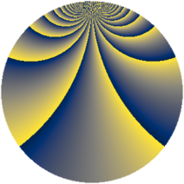# Properties

 Label 1425.2.boLevel $1425$ Weight $2$ Character orbit 1425.bo Rep. character $\chi_{1425}(224,\cdot)$ Character field $\Q(\zeta_{18})$ Dimension $696$ Sturm bound $400$

# Related objects

## Defining parameters

 Level: $$N$$ $$=$$ $$1425 = 3 \cdot 5^{2} \cdot 19$$ Weight: $$k$$ $$=$$ $$2$$ Character orbit: $$[\chi]$$ $$=$$ 1425.bo (of order $$18$$ and degree $$6$$) Character conductor: $$\operatorname{cond}(\chi)$$ $$=$$ $$285$$ Character field: $$\Q(\zeta_{18})$$ Sturm bound: $$400$$

## Dimensions

The following table gives the dimensions of various subspaces of $$M_{2}(1425, [\chi])$$.

Total New Old
Modular forms 1272 744 528
Cusp forms 1128 696 432
Eisenstein series 144 48 96

## Trace form

 $$696q + 12q^{4} - 12q^{6} + 12q^{9} + O(q^{10})$$ $$696q + 12q^{4} - 12q^{6} + 12q^{9} - 60q^{16} + 12q^{19} + 6q^{21} + 24q^{24} - 36q^{31} - 60q^{34} - 36q^{36} - 24q^{39} - 108q^{46} + 336q^{49} - 78q^{51} + 36q^{54} - 78q^{61} + 360q^{64} - 174q^{66} + 18q^{69} + 96q^{76} - 24q^{79} - 60q^{81} - 198q^{84} - 42q^{91} - 24q^{96} + 54q^{99} + O(q^{100})$$

## Decomposition of $$S_{2}^{\mathrm{new}}(1425, [\chi])$$ into newform subspaces

The newforms in this space have not yet been added to the LMFDB.

## Decomposition of $$S_{2}^{\mathrm{old}}(1425, [\chi])$$ into lower level spaces

$$S_{2}^{\mathrm{old}}(1425, [\chi]) \cong$$ $$S_{2}^{\mathrm{new}}(285, [\chi])$$$$^{\oplus 2}$$